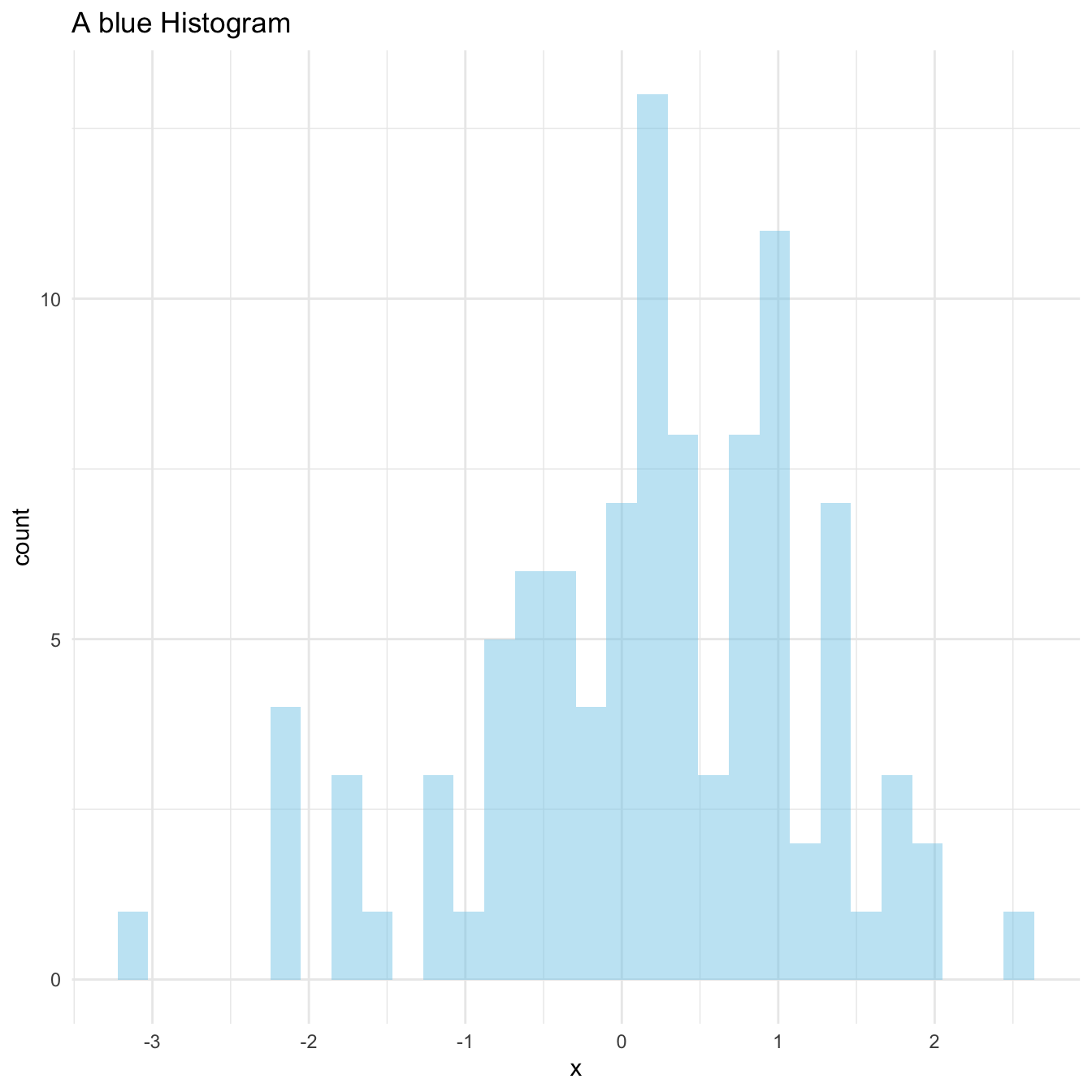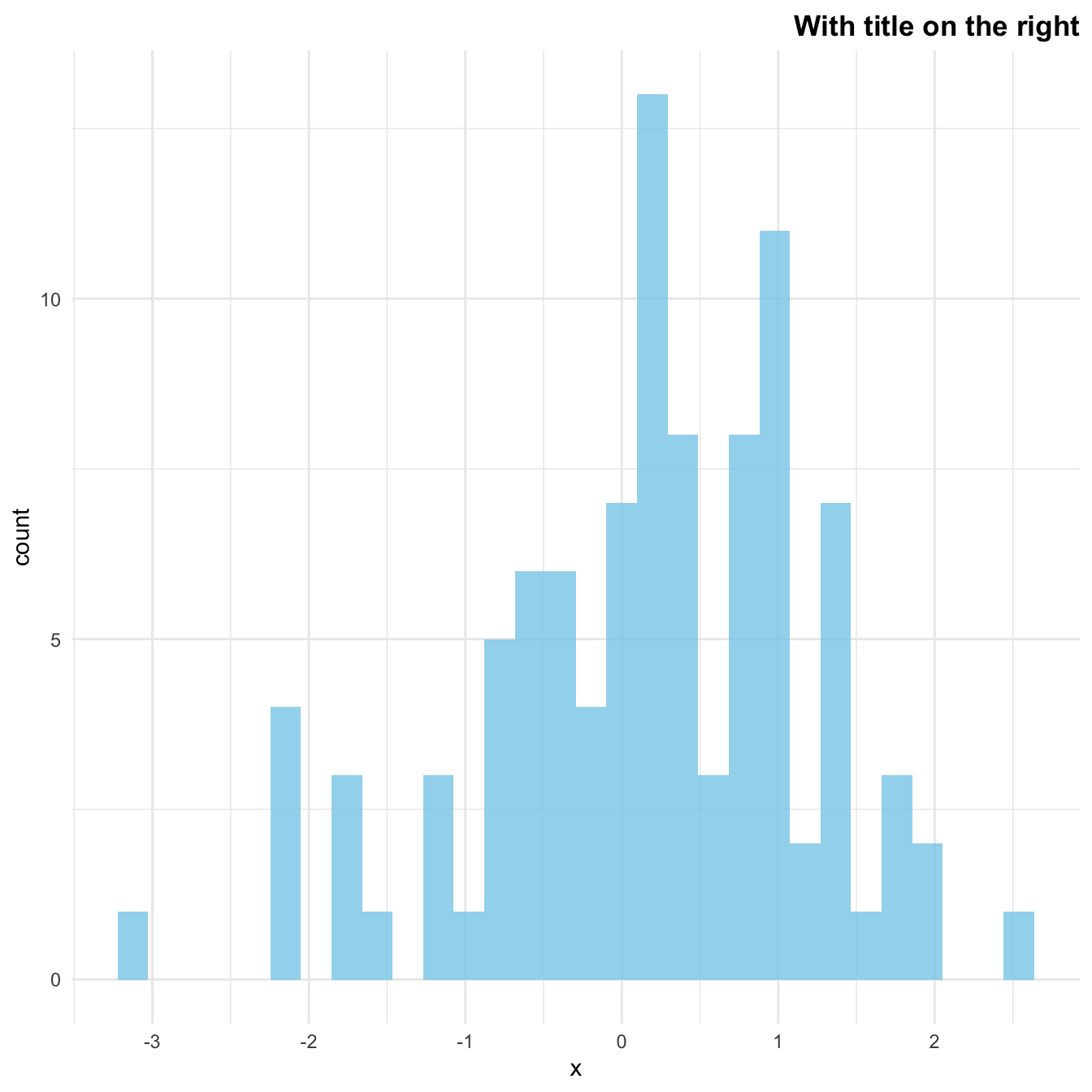# Title manipulation with R and ggplot2

This post describes all the available options to customize the chart title with `R` and `ggplot2`. It shows how to control its color, its position, and more.

# Default `ggplot2` title

It is possible to add a title to a ggplot2 chart using the `ggtitle()` function. It works as follow:``````# library & data
library(ggplot2)
data <- data.frame(x=rnorm(100))

# Basic plot with title
ggplot( data=data, aes(x=x)) +
geom_histogram(fill="skyblue", alpha=0.5) +
ggtitle("A blue Histogram") +
theme_minimal()``````

# Title on several lines

It is a common need to set the title on several lines. To add a break and skip to a second line, just add a `\n` in the text.``````# title on several lines
ggplot( data=data, aes(x=x)) +
geom_histogram(fill="skyblue", alpha=0.5) +
ggtitle("A blue \nHistogram") +
theme_minimal() ``````

# Title appearance and positionHere are 2 tricks to control text appearance and its position. Both features are controled thanks to the `plot.title` argument of the `theme()` function. Appearance can be controlled with option such as `family`, `size` or `color`, when position is controlled with `hjust` and `vjust`.

``````# Custom title appearance
ggplot( data=data, aes(x=x)) +
geom_histogram(fill="skyblue", alpha=0.5) +
ggtitle("A blue Histogram") +
theme_minimal() +
theme(
plot.title=element_text(family='', face='bold', colour='purple', size=26)
)``````
``````# Adjust the position of title
ggplot( data=data, aes(x=x)) +
geom_histogram(fill="skyblue", alpha=0.8) +
ggtitle("With title on the right") +
theme_minimal() +
theme(
plot.title=element_text( hjust=1, vjust=0.5, face='bold')
)``````

# Customize a specific word only

If you want to highlight a specific set of words in the title, it is doable thanks to the `expression()` function.``````# Custom a few word of the title only:
my_title <- expression(paste("A ", italic(bold("blue")), " histogram"))
ggplot( data=data, aes(x=x)) +
geom_histogram(fill="skyblue", alpha=0.8) +
ggtitle(my_title) +
theme_minimal()``````

Related chart types

## Contact

This document is a work by Yan Holtz. Any feedback is highly encouraged. You can fill an issue on Github, drop me a message on Twitter, or send an email pasting yan.holtz.data with gmail.com.